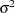Displayed Output

The GLM procedure produces the following output by default:

• The overall analysis-of-variance table breaks down the Total Sum of Squares for the dependent variable into the portion attributed to the Model and the portion attributed to Error.

• The Mean Square term is the Sum of Squares divided by the degrees of freedom (DF).

• The Mean Square for Error is an estimate of, the variance of the true errors.

• TheValue is the ratio produced by dividing the Mean Square for the Model by the Mean Square for Error. It tests how well the model as a whole (adjusted for the mean) accounts for the dependent variable’s behavior. Antest is a joint test to determine that all parameters except the intercept are zero.

• A small significance probability, Pr > F, indicates that some linear function of the parameters is significantly different from zero.

• R-Square,, measures how much variation in the dependent variable can be accounted for by the model., which can range from 0 to 1, is the ratio of the sum of squares for the model to the corrected total sum of squares. In general, the larger the value of, the better the model’s fit.

• Coeff Var, the coefficient of variation, which describes the amount of variation in the population, is 100 times the standard deviation estimate of the dependent variable, Root MSE (Mean Square for Error), divided by the Mean. The coefficient of variation is often a preferred measure because it is unitless.

• Root MSE estimates the standard deviation of the dependent variable (or equivalently, the error term) and equals the square root of the Mean Square for Error.

• Mean is the sample mean of the dependent variable.

These tests are used primarily in analysis-of-variance applications:

• The Type I SS (sum of squares) measures incremental sums of squares for the model as each variable is added.

• The Type III SS is the sum of squares for a balanced test of each effect, adjusted for every other effect.

These items are used primarily in regression applications:

• The Estimates for the model Parameters (the intercept and the coefficients)

• t Value is the Student’svalue for testing the null hypothesis that the parameter (if it is estimable) equals zero.

• The significance level, Pr > |t|, is the probability of getting a larger value ofif the parameter is truly equal to zero. A very small value for this probability leads to the conclusion that the independent variable contributes significantly to the model.

• The Standard Error is the square root of the estimated variance of the estimate of the true value of the parameter.

Other portions of output are discussed in the following examples.